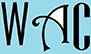## Calculating percentages; Solving equations & how long problems

This Webpage is meant to assist disadvantaged students in special education programs & special education with special needs and college students who need help with algebra strategies in order to successfully answer algebra questions. Below are strategies to popular algebra questions that they can work on or refer to in order to do their algebra homework or answer algebra problems on their algebra worksheets. These include: Calculating percentages; solving how many & how much questions; solving equations & how long problems.

These algebra questions below also have answers to the algebra questions when you click on each link. Here are a list of the most popular and most common answers to algebra questions that can be found in many algebra homework and algebra exams. The answers to algebra questions are explained in steps and the answers to algebra questions are easily understood. Below are questions, calculations, and answers for calculating percentages; solving how many & how much questions; solving equations & how long problems.